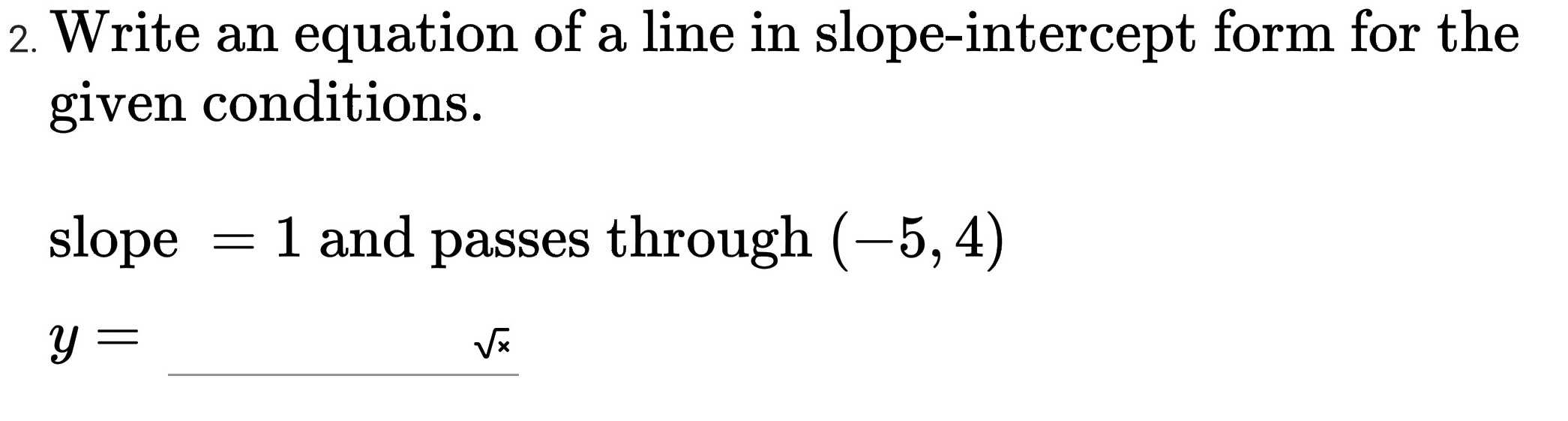### ¿Todavía tienes preguntas de matemáticas?

Pregunte a nuestros tutores expertos
Algebra
PreguntaWrite an equation of a line in slope-intercept form for the given conditions. slope $$= 1$$ and passes through $$( - 5,4 )$$

$$y =$$